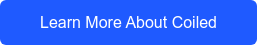# Parallelize pandas apply() and map() with Dask DataFrame

November 22, 2021

TLDR; Dask DataFrame can parallelize pandas apply() and map() operations, but it can do much more. With Dask’s map_partitions(), you can work on each partition of your Dask DataFrame, which is a pandas DataFrame, while leveraging parallelism for various custom workflows.

This data is stored in a public bucket and you can use Coiled for free if you’d like to run these computations yourself.Follow along with the code in this notebook!

Dask DataFrame helps you quickly scale your single-core pandas code, while keeping the API familiar. The map() and apply() functions are at the core of data manipulation with pandas. In this post, we’ll take a closer look at how you can perform these operations efficiently with Dask DataFrame. We’ll also look at some additional features that Dask provides to level-up your computations.

In particular,

• Dask’s map_partitions() function, and
• Dask’s implementation of pandas apply(), map(), and applymap().

## Quick overview of pandas apply() and map()

You can use pandas’ apply() function to apply any in-built or custom Python function across a pandas one-dimensional array, i.e., a Series or a single dimension of a DataFrame. This can be applied across columns (axis=0), or rows (axis=1).

Consider a pandas DataFrame ‘df’ with 10 rows and 2 columns: ‘a’ and ‘b’, where each element in the DataFrame is a random integer:

Also, consider a function minmax() that sleeps for 1 second and returns the difference between the largest and smallest value:

```def minmax(x):
sleep(1)
return x.max() - x.min()```

We’ll use apply() to do the minmax() operation on all the rows in df:

`df.apply(minmax, axis=1)`

This computation takes ~10s because we have 10 rows. (We’re noting the time to compare it against Dask later!)

You can use the map() and applymap() functions for element-wise operations across a pandas Series and DataFrame respectively.

Consider a function ‘inc’ that sleeps for 1s and returns the input incremented by 1:

```def inc(i):
sleep(1)
return i+1```

We’ll use map() to do this inc() operation across a column in the pandas DataFrame (which is a pandas Series):

`df.a.map(inc)`

And then, across the entire dataframe using applymap():

`df.applymap(inc)`

We noted the time for these operations as well, the Series map took ~10 seconds and DataFrame applymap took ~20 seconds.## Dask Map Partitions

A Dask DataFrame consists of multiple pandas Dataframes, and each pandas dataframe is called a partition. This mechanism allows you to work with larger-than-memory data because your computations are distributed across these pandas dataframes and can be executed in parallel. This includes our apply() and map() computations!

First, we need to convert our pandas dataframe into a dask dataframe ‘ddf’:

`ddf = dd.from_pandas(df, npartitions=5)  # Dask DataFrame has 5 partitions`

We can manually access and work on these individual partitions using Dask’s map_partitions() function. map_partitions() maps any function across each partition, so you can operate on each partition as you would on a pandas DataFrame.

We can define a new function called minmax2 that operates on a pandas DataFrame and applies the previous minmax function on the DataFrame:

```def minmax2(df):
return df.apply(minmax, axis=1)```

Now, we’ll use map_partitions() to map this function minmax2 across ddf:

`ddf.map_partitions(minmax2, meta=(None, 'int64'))`

The visualization below shows how minmax2 was mapped across all 5 partitions of ddf, and hence, was almost 5x as fast as the original pandas apply().

In the visualizations, rectangular nodes represent Python objects, circular nodes represent functions, and the arrows between them represent task dependencies.

## Meta keyword argument

map_partitions() and all the functions mentioned below have an important keyword argument called ‘meta’. It describes the structure of the expected output of your computation. These functions are very flexible and can present many different types of outputs, which is why the ‘meta’ argument is essential.

It is an optional argument. If it’s not provided, Dask DataFrame tries to infer the output type with a sample input (which can also take some time), but it’s good practice to describe it yourself. You can describe ‘meta’ as a pandas DataFrame, pandas Series, Python dictionary, Python iterable, or a Python tuple.

## Dask’s apply() and map()

Dask also implements the pandas apply(), map(), and applymap() functions which (similar to map_partitions()) operate on each partition of the dataframe in a parallel fashion. Let’s look at some examples below:

`q = ddf.apply(minmax, axis=1, meta=(None, 'int64'))`

Dask DataFrame Series map:

`r = ddf.a.map(inc)`

`s = ddf.applymap(inc)`

##Notice how these computations are ~5x as fast as the corresponding pandas operations!

## map_partitions() vs apply()

We have used the pandas and Dask apply() functions in this blog post only for understanding purposes. In actual use cases, it’s not a good idea to use apply() with custom Python code because of its slow performance. We recommend using map_partitons() instead.

If you’d like to scale your Dask work to the cloud, check out Coiled — Coiled provides quick and on-demand Dask clusters along with tools to manage environments, teams, and costs. Click below to learn more!